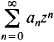# Radius of Convergence

(redirected from Disc of convergence)

## radius of convergence

[′rād·ē·əs əv kən′vər·jəns]
(mathematics)
The positive real number corresponding to a power series expansion about some number a with the property that if x-a has absolute value less than this number the power series converges at x, and if x-a has absolute value greater than this number the power series diverges at x.

## Radius of Convergence

The radius of convergence of a power series is the radius of the circle of convergence. In other words, it is the number r such that the power seriesconverges when ǀzǀ > r and diverges when ǀzǀ > r.

Site: Follow: Share:
Open / Close